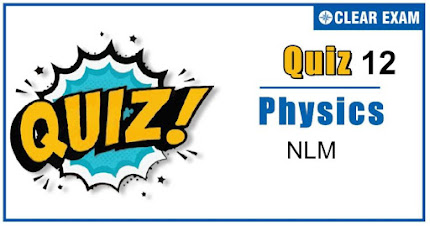## [LATEST]\$type=sticky\$show=home\$rm=0\$va=0\$count=4\$va=0

As per analysis for previous years, it has been observed that students preparing for NEET find Physics out of all the sections to be complex to handle and the majority of them are not able to comprehend the reason behind it. This problem arises especially because these aspirants appearing for the examination are more inclined to have a keen interest in Biology due to their medical background. Furthermore, sections such as Physics are dominantly based on theories, laws, numerical in comparison to a section of Biology which is more of fact-based, life sciences, and includes substantial explanations. By using the table given below, you easily and directly access to the topics and respective links of MCQs. Moreover, to make learning smooth and efficient, all the questions come with their supportive solutions to make utilization of time even more productive. Students will be covered for all their studies as the topics are available from basics to even the most advanced. .

Q1. A force of 10 Newton acts on a body of mass 20 kg for 10 seconds. Change in its momentum is:
•  5 kgm/s
•  100 kgm/s
•  200 kgm/s
•  1000 kgm/s
Solution
dp=F×dt=10×10=100 kgm/s

Q2. A man weighs 80 kg. He stands on a weighing scale in a lift which is moving upwards with a uniform acceleration of 5 m/s2. What would be the reading on the scale. (g=10 m/s2):
•  400 N
•  800 N
•  1200 N
•  Zero
Solution
For accelerated upward motion R=m(g+a)=80 (10+5)=1200 N .

Q3. The coefficient of kinetic friction between a 20 kg box and the floor is 0.40. How much work does a pulling force do on the box in pulling it 8.0 m across the floor at constant speed? The pulling force is directed 37° above the horizontal:
•   343 J
•  482 J
•  14.4 J
•  None of these

Q4. In the arrangement shown in figure, if a force 2 mg is applied at the free end of the rope, the mass m will ascend with an acceleration of

•  g/3
•  g/2
•  g
•  2 g
Solution
Force 2mgapplied at the free end of the string acts on mass m. Therefore, its acceleration a=Force/mass =2mg/m=2g

Q5.  A vessel containing water is given a constant acceleration a towards the right, along a straight horizontal path. Which of the following diagram represents the surface of the liquid

•  A
•  B
•  C
•  D
Solution
Due to acceleration in forward direction, vessel is in an accelerated frame therefore a Pseudo force will be exerted in backward direction. Therefore water will be displaced in backward direction

Q6. Two masses m_1=1kg and m_2=2kg are connected by a light inextensible string and suspended by means of a weightless pulley as shown in figure.

Assuming that both the masses start from rest, the distance travelled by the centre of mass in 2 s is (take g=10m/s2)
•  20/9 m
•  40/9 m
• 2/3 m
•  1/3 m
Q7.  A body is coming with a velocity of 72 kmh-1 on a rough horizontal surface of coefficient of friction 0.5. If the acceleration due to gravity is 10 ms-2, find the minimum distance it can be stopped:
•  400 m
•  40 m
•  0.40 m
•  4 m
Solution
Velocity u=72 kmh-1 =20 ms-1 a= μg=0.5×10 ms-2 From v2=u2-2as ∴ (0)2=(20)2-2×0.5×10×s .

Q8.  A block B is pushed momentarily along a horizontal surface with an initial velocity V. If μ is the coefficient of sliding friction between B and the surface, block B will come to rest after a time:

•  V/(gμ)
•  Tgμ/V
•  g/V
•  V/g
Solution
Friction is the retarding force for the block F=ma=μR=μmg Retardation a=μg From first equation to motion v=u-at ⇒0=V-μg×t⇒t=V/gμ .

Q9.  A mass of 6 kg is suspended by a rope of length 2 m from a ceiling. A force of 50 N is applied in the horizontal direction at the mid-point of the rope. The angel made by the rope, with the vertical, in equilibrium position will be (take g=10 ms-2), neglect the mass of the rope)
•  90°
•  60°
•  50°
•  40°
Solution
The three forces acting on the mass at location A have been shown in figure. Since the mass is in equilibrium, therefore, the three forces acting on the mass must be represented by the three sides of a triangle taken in one order. Hence

Q10. The average force necessary to stop a bullet of mass 20 g moving with a speed of 250 m/s, as it penetrates into the wood for a distance of 12 cm is:
•  2.2×103 N
•  3.2×103 N
•  4.2×103 N
• 5.2×103 N

∴F=5.2×103 N## Want to know more

Please fill in the details below:

## Latest NEET Articles\$type=three\$c=3\$author=hide\$comment=hide\$rm=hide\$date=hide\$snippet=hide

Name

ltr
item
BEST NEET COACHING CENTER | BEST IIT JEE COACHING INSTITUTE | BEST NEET & IIT JEE COACHING: NLM Quiz-12
NLM Quiz-12
https://1.bp.blogspot.com/-6hyxGemqKJs/X4vc2ZrsCBI/AAAAAAAAAKA/1UftC-IkrxoDBbkQuxiX9T01Ve-vo9xwACLcBGAsYHQ/w430-h226/Quiz%2BImage%2BTemplate%2B%252811%2529.jpg
https://1.bp.blogspot.com/-6hyxGemqKJs/X4vc2ZrsCBI/AAAAAAAAAKA/1UftC-IkrxoDBbkQuxiX9T01Ve-vo9xwACLcBGAsYHQ/s72-w430-c-h226/Quiz%2BImage%2BTemplate%2B%252811%2529.jpg
BEST NEET COACHING CENTER | BEST IIT JEE COACHING INSTITUTE | BEST NEET & IIT JEE COACHING
https://www.cleariitmedical.com/2020/10/NLM%20Quiz-12.html
https://www.cleariitmedical.com/
https://www.cleariitmedical.com/
https://www.cleariitmedical.com/2020/10/NLM%20Quiz-12.html
true
7783647550433378923
UTF-8

STAY CONNECTED